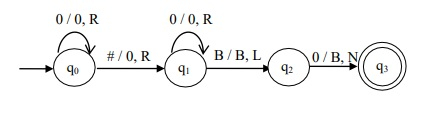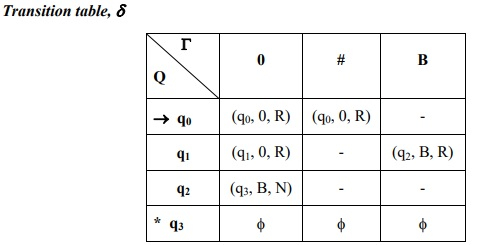# Design a TM to compute addition of two unary numbers

Data Structure AlgorithmsComputer ScienceComputers

#### Big Data Hadoop

Best Seller

89 Lectures 11.5 hours

#### Practical Data Science using Python

22 Lectures 6 hours

#### Data Science and Data Analysis with Python

50 Lectures 3.5 hours

The unary input number n is represented with a symbol 0 n – times.

## Example

• 4 → 0000

• 1 → 0

• 5 → 00000

The separation symbol, „#‟ (any other special character) shall be used to distinguish between two or more inputs.

For Example: 5, 2 are the inputs represented by 00000 # 00.

## Algorithm

Step 1 - Read the symbols of the first input with no replacements and move right.

Step 2 - When the symbol = ‘#’, replace it by ‘0’ and move right.

Step 3  - Traverse right side until the rightmost ‘0’ (left to B – last symbol)

Step 4 - Replace the rightmost ‘0’ by B

Step 5 - Stop the machine.

## Turing Machine

The Turing Machine (TM) is as follows −The Turing machine, M is given by M = (Q, ∑, Γ, δ, q0, B, F)

Where,

• Q = {q0, q1, q2, q3}

• Σ = {0, #}

• Γ = {0, #, B}

• δ ⇒ Given by the above transition diagram q

• 0 = {q0}

• B = {B}

• F = {q3}